# f. Demonstration polymorphic behavior by declaring a Point2D pointer and separately assigning a Point2D object then...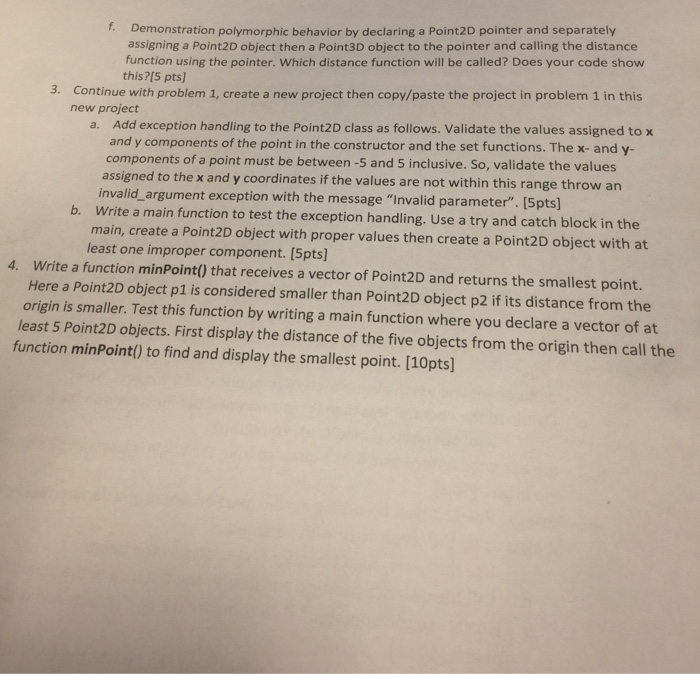f. Demonstration polymorphic behavior by declaring a Point2D pointer and separately assigning a Point2D object then a Point3D object to the pointer and calling the distance function using the pointer. Which distance function will be called? Does your code show this?[5 pts) Continue with problem 1, create a new project then copy/paste the project in problem 1 in this new project 3. a. Add exception handling to the Point2D class as follows. Validate the values assigned to x and y components of the point in the constructor and the set functions. The x- and y- components of a point must be between -5 and 5 inclusive. So, validate the values assigned to the x and y coordinates if the values are not within this range throw an invalid_argument exception with the message "Invalid parameter". [Spts Write a main function to test the exception handling. Use a try and catch block in the b. main, create a Point2D object with proper values then create a Point2D object with at least one improper component. [5pts] 4. Write a function minPoint() that receives a vector of Point2D and returns the smallest point. Here a Point2D object p1 is considered smaller than Point2D object p2 if its distance from the origin is smaller. Test this function by writing a main function where you declare a vector of at east 5 Point2D objects. First display the distance of the five objects from the origin then call the function minPoint() to find and display the smallest point. [10pts)]

C++ Code:

(4)

#include <iostream>
#include <vector>

using namespace std;

//Point class definition
class Point2D
{
//Private member variables
private:
double XCord, YCord;

//Public methods
public:

//Default Constructor
Point2D()
{
XCord = 0;
YCord = 0;
}

//Constructor that has 2 parameters(x coordinate , y coordinate)
Point2D(double x, double y)
{
//Setting x,y values
XCord = x;
YCord = y;
}

//Function that sets X Co-ordinate
void setXCord(double x)
{
XCord = x;
}

//Function that sets Y Co-ordinate
void setYCord(double y)
{
YCord = y;
}

//Function that returns X Co-ordinate
double getXCord()
{
return XCord;
}

//Function that returns Y Co-ordinate
double getYCord()
{
return YCord;
}

//Function that returns distance from point to origin
double distance()
{
double dist;

//Calculating distance d = sqrt( x^2 + y^2 )
dist = sqrt( (double)(XCord*XCord) + (double)(YCord*YCord) );

//Return distance
return dist;
}

friend ostream &operator<<( ostream &output, const Point2D &D)
{
//Forming a result statement
output << "(" << D.XCord << ", " << D.YCord << ")";
return output;
}
};

bool operator<(Point2D p1, Point2D p2)
{
//Comparing distances
if(p1.distance() < p2.distance())
{
return true;
}
return false;
}

//Function midpoint
void midPoint(vector<Point2D> points)
{
int smallest;

//Initially assume that starting point is the smallest point
smallest = 0;

//Iterating over vector
for(int i=0; i<points.size(); i++)
{
//Comparing points
if(points.at(i) < points.at(smallest))
{
//Updating smallest point
smallest = i;
}
}

//Displaying smallest point
cout << "\n\n Smallest Point: " << points.at(smallest) << " \n\n";
}

//Main method
int main()
{
Point2D point1(6.2, 4.8);
Point2D point2(3.6, 5.9);
Point2D point3(8.6, 1.3);
Point2D point4(6.5, 2.4);
Point2D point5(10.2, 1.2);

//Creating a vector
vector<Point2D> points;

points.push_back(point1);
points.push_back(point2);
points.push_back(point3);
points.push_back(point4);
points.push_back(point5);

//Iterating over vector
for(int i=0; i<points.size(); i++)
{
cout << "\n\n Distance of Point " << (i+1) << " " << points.at(i) << " from origin: " << (points.at(i)).distance();
}

cout << "\n\n\n Midpoint: ";

//Calling midpoint method
midPoint(points);

return 0;
}

____________________________________________________________________________________________

Sample Run: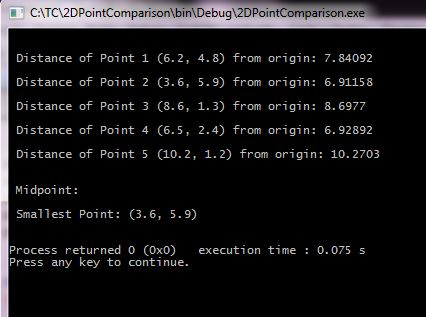#### Earn Coin

Coins can be redeemed for fabulous gifts.

Similar Homework Help Questions
• ### a) (10 pts) Create a class called Cartesian that contains two double members, x and y....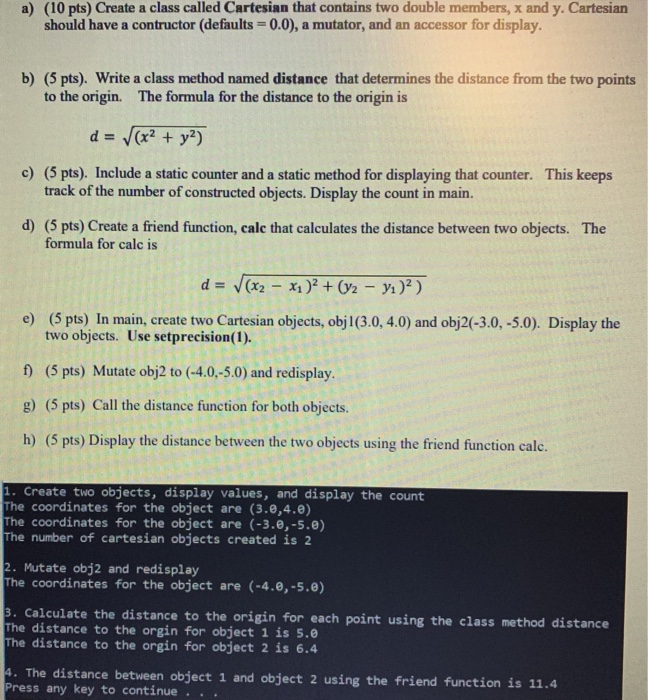a) (10 pts) Create a class called Cartesian that contains two double members, x and y. Cartesian should have a contructor (defaults = 0.0), a mutator, and an accessor for display. b) (5 pts). Write a class method named distance that determines the distance from the two points to the origin. The formula for the distance to the origin is d = (x2 + y2) c) (5 pts). Include a static counter and a static method for displaying that counter....

• ### This is my code that i need to finish. In BoxRegion.java I have no idea how...

This is my code that i need to finish. In BoxRegion.java I have no idea how to create the constructor. I tried to use super(x,y) but It is hard to apply. And Also In BoxRegionHashTable, I don't know how to create displayAnnotation BoxRegion.java ------------------------------------------------ public final class BoxRegion { final Point2D p1; final Point2D p2; /** * Create a new 3D point with given x, y and z values * * @param x1, y1 are the x,y coordinates for point...

• ### The purpose of this is to use inheritance, polymorphism, object comparison, sorting, reading binary files, and...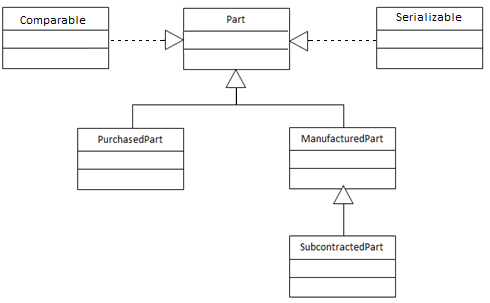The purpose of this is to use inheritance, polymorphism, object comparison, sorting, reading binary files, and writing binary files. In this application you will modify a previous project. The previous project created a hierarchy of classes modeling a company that produces and sells parts. Some of the parts were purchased and resold. These were modeled by the PurchasedPart class. Some of the parts were manufactured and sold. These were modeled by the ManufacturedPart class. In this you will add a...

• ### question

: Implement the following given scenario in C++ a. Create an inheritance hierarchy by writing the source code, containing base class BankAccount and derived classes SavingsAccount and CheckingAccount that inherit from class BankAccount. b. Implement Polymorphism where required to achieve the polymorphic behavior. c. Multiple constructors be defined for initializing the account of a user. d. The class should provide four member functions. i. Member function Credit should add an amount to the current balance. ii. Member function Debit should...

• ### I am having trouble understanding how this code is able to use the contents of the...

I am having trouble understanding how this code is able to use the contents of the header file. Can someone please provide comments in the main code to describe what is happening? (especially on the bool isNumber) THE MAIN CODE: #include<bits/stdc++.h> #include "MyCartesianPoint.h" #include <math.h> #include <iostream> using namespace std; bool isNumber(string s) {    if(!isdigit (s))    {        if(s != '-')        return false;               else if(s.length() == 1)        return false;...

• ### Hello I need help with this program. Should programmed in C! Program 2: Sorting with Pointers...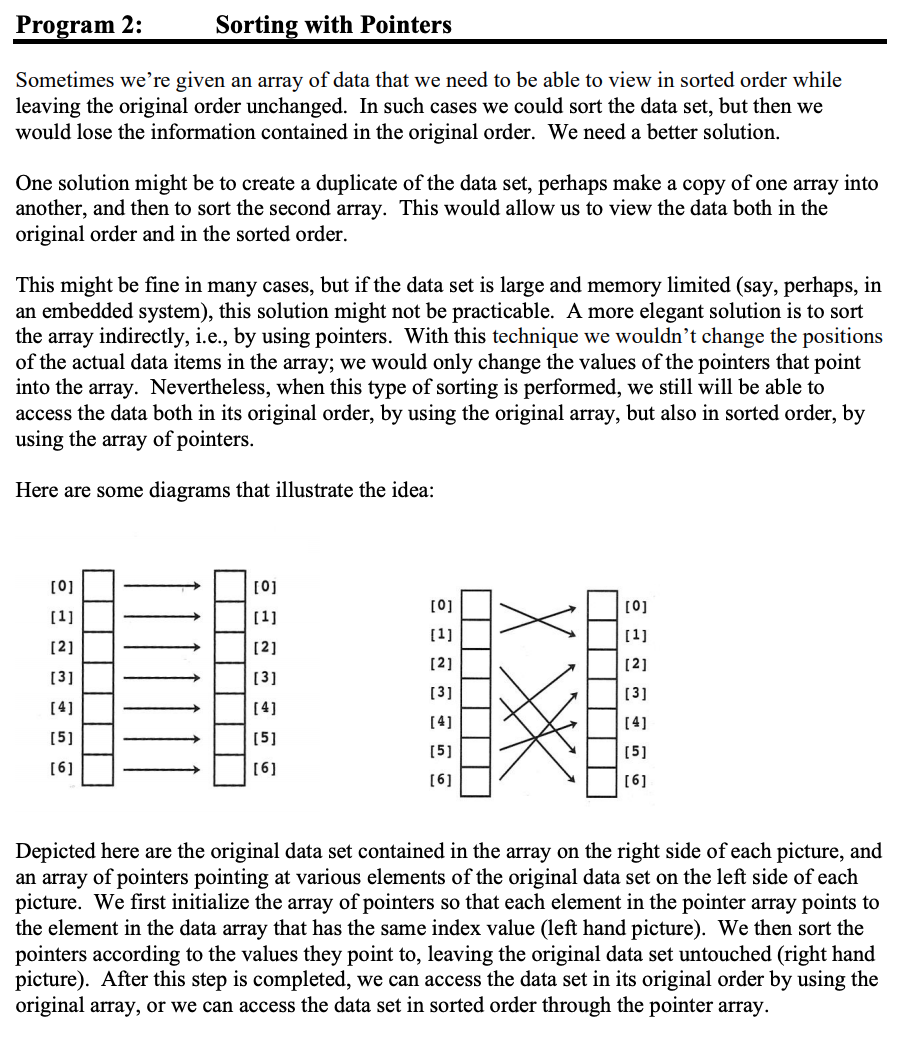Hello I need help with this program. Should programmed in C! Program 2: Sorting with Pointers Sometimes we're given an array of data that we need to be able to view in sorted order while leaving the original order unchanged. In such cases we could sort the data set, but then we would lose the information contained in the original order. We need a better solution. One solution might be to create a duplicate of the data set, perhaps make...

• ### please there are some specific instructions on the question so i would greatly appreciate if they...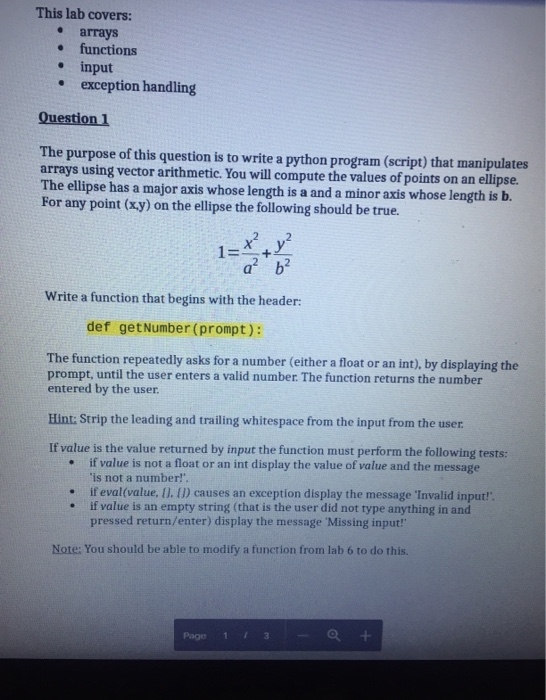please there are some specific instructions on the question so i would greatly appreciate if they are followed . thank you very much for your time This lab covers: arrays functions input exception handling Question 1 The purpose of this question is to write a python program (script) that manipulates arrays using vector arithmetic. You will compute the values of points The ellipse has a major axis whose length is a and a minor axis whose length is b. For...

• ### Dynamic Lists: Pointer Issues

I have been working in Visual C++ 2008 &puTTY to figure out how to make this work. puTTY keeps giving me segmentation errors; Visual C++ gives me thefollowing:"Unhandled exception at 0x10296af2 in ForProj3.exe: 0xC0000005: Access violation writing location 0x00000000."(Note: select 5 at the switch statement)Here is where Visual C++ brings me to (lines 177-179, I tried to flag in the code text below; extra comments were removed):fscanf(dataPtr, "%d",aCtr);printf("%d read from filen",aCtr);AddToList( &sPtr, aCtr );What is causing the segmentation error? I'm...

• ### Program Purpose In this program you will demonstrate your knowledge in programming OOP concepts, such as classes, encapsulation, and procedural programming concepts such as lınked lists, dynamic me...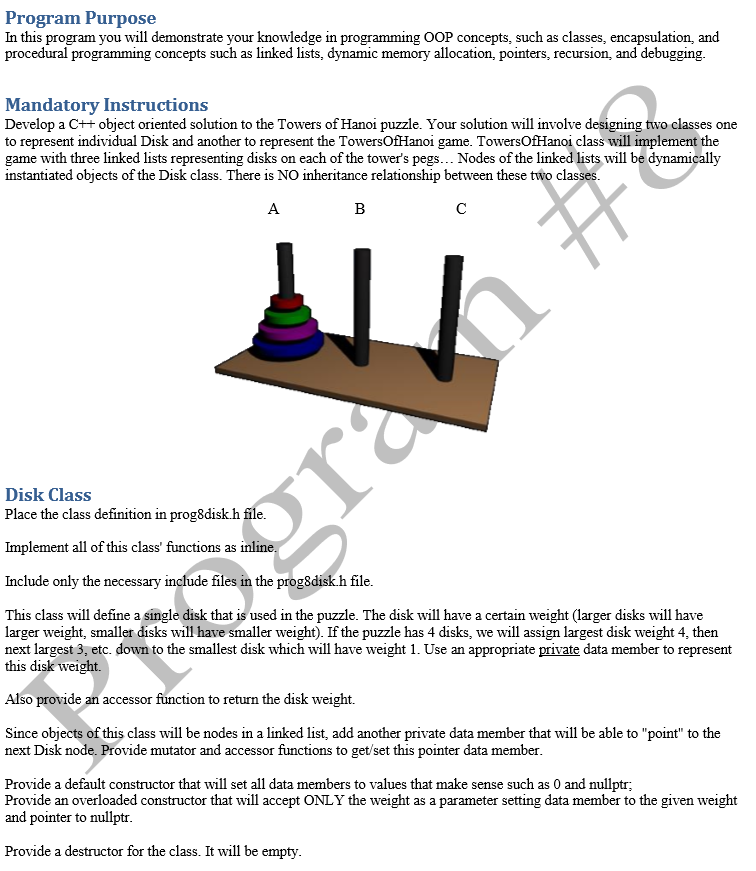Program Purpose In this program you will demonstrate your knowledge in programming OOP concepts, such as classes, encapsulation, and procedural programming concepts such as lınked lists, dynamic memory allocation, pointers, recursion, and debugging Mandatory Instructions Develop a C++ object oriented solution to the Towers of Hanoi puzzle. Your solution will involve designing two classes one to represent individual Disk and another to represent the TowersOfHanoi game. TowersOfHanoi class will implement the game with three linked lists representing disks on each...

• ### Write a MATLAB Graphical User Interface (GUI) to simulate and plot the projectile motion – the...

Write a MATLAB Graphical User Interface (GUI) to simulate and plot the projectile motion – the motion of an object projected into the air at an angle. The object flies in the air until the projectile returns to the horizontal axis (x-axis), where y=0. This MATLAB program should allow the user to try to hit a 2-m diameter target on the x-axis (y=0) by varying conditions, including the lunch direction, the speed of the lunch, the projectile’s size, and the...